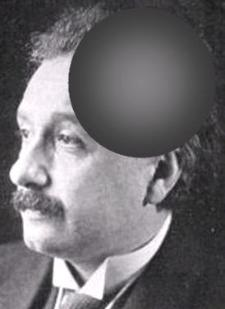A mathematician says that solids are hollowAdapted for the Internet from:Why God Doesn't Exist

Summary

Of all the geometric figures, none causes so much trouble to a mathematician than a sphere.
A sphere is the only solid known to man that has four different dimensions. According to the
mathematicians, a sphere may be 1-D, 2-D, 3-D, or 4-D; it all depends on how many beers
they've had at the pub that day. A mathematician uses two or more of these definitions during
a presentation just to make sure he covered all the bases and made his theory unfalsifiable.

But I'm really joking. The sphere of Mathematics is really two-dimensional. In the religion of
Mathematical Physics, the sphere is the only solid which is not a solid.

The differences with the sphere of Physics don't stop there.

The sphere of Mathematics is hollow like a mathematician's skull.
The sphere of Physics, as its category suggests, is solid: like a bowling ball.
The sphere of Math is rough, like the mastery a mathematician has of language.
The sphere of Physics is ideally smooth.
The sphere of Math is still under construction (The Inconclusive Sphere perhaps?).
It is a movie that the director promises to finish someday.
The sphere of Physics is ready to be served.

Physics is so different from Math that you wonder what the idiots of Math are talking about
when they say that Math is the language of Physics.The hollow sphere of Mathematics________________________________________________________________________________________